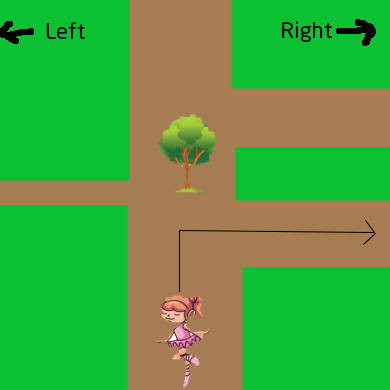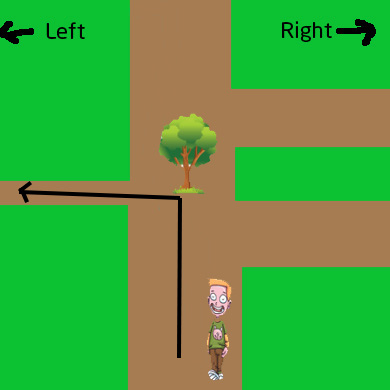1. Based on the cubes or number of sticks shown, can you tell what number is shown. Count by tens and then ones.

Tens   Ones

2.Write the number shown below. Add the tens and then ones.

3Draw number '77' on the numbers line below.

4. Solve:

80 + 1 =

5. Solve:

5 tens + 1 ones =

6.Use the tens frames to help you find the answer to the problem shown on the right.

8 + 4 =

7.Use the tens frames to help you find the answer to the problem shown on the right.

20 - 1 =
Copyright SubjectCoach.com. Strictly for Personal and School Use Only.

8.Use the number line to answer the question below.

6 + 2 =

9.Use the number line to answer the question below.

10 - 2 =

10.Find the missing number in the sequence shown below

25,        , 21, 19, 17

11.Find the missing number in the sequence shown below

85, 80, 75,        , 65

12.Find the missing number in the sequence shown below

2, 12, 22,        , 42

13.Shade one half of the shape shown below

14. Check the more valuable coin.

15. Check the more valuable coin.

Copyright SubjectCoach.com. Strictly for Personal and School Use Only.

16.How much do I have?

17. Complete the pattern.

57, 58,       , 60,       , 62, 63,

18. Complete the pattern.

40,       ,       , 55, 60, 65, 70,

19. Complete the sequence shown below by fill in the blanks

, 90, 80, 70,       ,       , 40, 30

20. What time is shown on the clock

To

21. What time is shown on the clock

To

22. How many days of the week don't start with S or T?

23. How many corners does a square have?

Copyright SubjectCoach.com. Strictly for Personal and School Use Only.

24. Ben and Sally were asked to turn right before reaching the tree. The path they took is shown. Who followed the directions correctly?25. What's the chance that you will sneeze tomorrow?
Write your answer by choosing one of these options: won't happen,will happen,might happen

26. Allie went to the farmers’ market and saw 8 oranges, 2 pears, and 7 apples. Can you make a bar graphs from this data below.

Copyright SubjectCoach.com. Strictly for Personal and School Use Only.
##### -- Intentionally left blank --

1. Based on the cubes or number of sticks shown, can you tell what number is shown. Count by tens and then ones.

Tens   Ones

2.Write the number shown below. Add the tens and then ones.

3Draw number '77' on the numbers line below.

4. Solve:

80 + 1 =

5. Solve:

5 tens + 1 ones =

6.Use the tens frames to help you find the answer to the problem shown on the right.

8 + 4 =

7.Use the tens frames to help you find the answer to the problem shown on the right.

20 - 1 =
Copyright SubjectCoach.com. Strictly for Personal and School Use Only.

8.Use the number line to answer the question below.

6 + 2 =

9.Use the number line to answer the question below.

10 - 2 =

10.Find the missing number in the sequence shown below

25,   23  , 21, 19, 17

11.Find the missing number in the sequence shown below

85, 80, 75,   70  , 65

12.Find the missing number in the sequence shown below

2, 12, 22,   32  , 42

13.Shade one half of the shape shown below

14. Check the more valuable coin.

15. Check the more valuable coin.

Copyright SubjectCoach.com. Strictly for Personal and School Use Only.

16.How much do I have?

17. Complete the pattern.

57, 58,   59  , 60,   61  , 62, 63,   64

18. Complete the pattern.

40,   45  ,   50  , 55, 60, 65, 70,   75

19. Complete the sequence shown below by fill in the blanks

100  , 90, 80, 70,   60  ,   50  , 40, 30

20. What time is shown on the clock

To

21. What time is shown on the clock

To

22. How many days of the week don't start with S or T?

3 days

23. How many corners does a square have?

4 corners

Copyright SubjectCoach.com. Strictly for Personal and School Use Only.

24. Ben and Sally were asked to turn right before reaching the tree. The path they took is shown. Who followed the directions correctly?Sally

25. What's the chance that you will sneeze tomorrow?
Write your answer by choosing one of these options: won't happen,will happen,might happen

might happen

26. Allie went to the farmers’ market and saw 8 oranges, 2 pears, and 7 apples. Can you make a bar graphs from this data below.

Copyright SubjectCoach.com. Strictly for Personal and School Use Only.